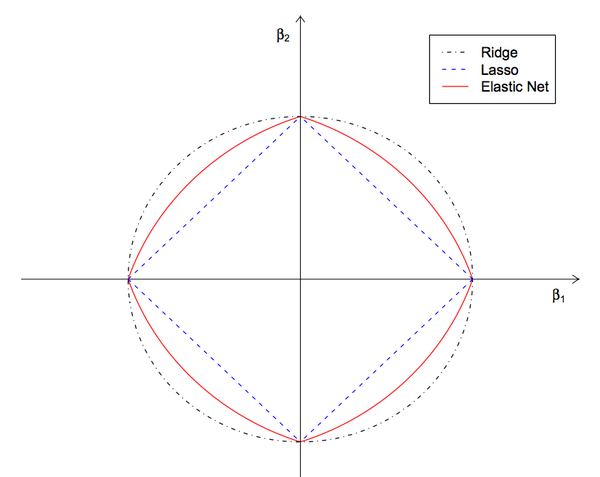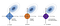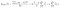# ElasticNet Regression Fundamentals and Modeling in Python

In this blog post, I will first try to explain the basics of ElasticNet Regression. Then, we’ll build the model using a dataset with Python. Finally, we’ll evaluate the model by calculating the mean square error. Let’s get started step by step.Resource: https://www.oreilly.com/library/view/machine-learning-with/9781787121515/5c5ec380-d139-49a5-99b1-3ce32ae5bd6f.xhtml

# What is the ElasticNet Regression?

The main purpose of ElasticNet Regression is to find the coefficients that minimize the sum of error squares by applying a penalty to these coefficients. ElasticNet combines L1 and L2 (Lasso and Ridge) approaches. As a result, it performs a more efficient smoothing process. In another source, it is defined as follows:

Elastic Net first emerged as a result of critique on Lasso, whose variable selection can be too dependent on data and thus unstable. The solution is to combine the penalties of Ridge regression and Lasso to get the best of both worlds.

• It combines the L1 and L2 approaches.
• It performs a more efficient regularization process.
• It has two parameters to be set, λ and α.

The elastic net method improves on lasso’s limitations, i.e., where lasso takes a few samples for high dimensional data, the elastic net procedure provides the inclusion of “n” number of variables until saturation. In a case where the variables are highly correlated groups, lasso tends to choose one variable from such groups and ignore the rest entirely.Differences between L1, L2, and L1+L2 Norm

# ElasticNet Regression Model

Elastic Net aims at minimizing the following loss function:ElasticNet Mathematical Model

The terms used in the mathematical model are the same as in Ridge and Lasso Regression. That’s why I won’t go back to it here. You can review the explanations about the mathematical model from the articles I shared below.

Ridge Regression

Lasso Regression

# Modeling with Python

Now let’s build a `ElasticNet Regression` model on a sample data set. And then let’s calculate the square root of the model’s `Mean Squared Error` This will give us the model error.

First of all, we import the libraries necessary for modeling as usual.

Then we do data reading and some data editing operations.

With ElasticNet regression, we set up the model on the train set.

I do not go into concept details such as what is fit, what is a train set.

According to the variables in the data set we have, we find the variable coefficients in the ElasticNet model as follows.

We found the constant of the ElasticNet regression model to be -6,46 with the following function.

Now let’s make the model prediction under normal conditions without specifying any parameters. We can see the first 10observations of the model prediction for the train set as follows.

Likewise, we can see the first 10observations of the model prediction for the test set as follows.

Then we saved the values we predicted over the test set in a cluster named y_pred. And we found the RMSE value as 357,16 as a result of the following calculation.

As a result, we found the R-Squared score as 0,41. The R-squared score is the percentage of the change in the dependent variable explained by the independent variables.

In other words, we can say that independent variables in ElasticNet Regression Model explain 41.07% of the change in dependent variables for this data set.

What is R-Squared?

R-squared (R2) is a statistical measure that represents the proportion of the variance for a dependent variable that’s explained by an independent variable or variables in a regression model. Whereas correlation explains the strength of the relationship between an independent and dependent variable, R-squared explains to what extent the variance of one variable explains the variance of the second variable. So, if the R2 of a model is 0.50, then approximately half of the observed variation can be explained by the model’s inputs.

In this section, we will do the operations using the ElasticNetCV method to find the optimum lambda value.

Accordingly, we find the alpha value as 5230,76.

Afterward, we can find the constant of the model established with ElasticNetCV as follows.

We can find the coefficients of the variables of the model established with ElasticNetCV as follows.

Then we rebuild the Adjusted ElasticNet model with this optimum alpha value. Then we print the predicted values from the test set into y_pred. As a result, we find the RMSE value as 394,15.

# Finally

First, we examined what is ElasticNet Regression in this blog post. Then we talked about the features and basics of ElasticNet Regression. Mathematically, we examined the model of this algorithm. Then we set up the model under the current conditions and calculate the error value. In the Model Tuning part, we calculated the corrected error value by calculating the optimum alpha value with ElasticNetCV and rebuilding the corrected model according to this alpha value.

# Resources

## MLearning.ai

Data Scientists must think like an artist when finding a solution

## MLearning.ai

Data Scientists must think like an artist when finding a solution, when creating a piece of code.Artists enjoy working on interesting problems, even if there is no obvious answer.

Written by

## Kerem Kargın

BSc Industrial Eng. | #MachineLearning #ComputerVision #DeepLearning | BI Developer https://www.linkedin.com/in/keremkargin/## MLearning.ai

Data Scientists must think like an artist when finding a solution, when creating a piece of code.Artists enjoy working on interesting problems, even if there is no obvious answer.

Medium is an open platform where 170 million readers come to find insightful and dynamic thinking. Here, expert and undiscovered voices alike dive into the heart of any topic and bring new ideas to the surface. Learn more

Follow the writers, publications, and topics that matter to you, and you’ll see them on your homepage and in your inbox. Explore

If you have a story to tell, knowledge to share, or a perspective to offer — welcome home. It’s easy and free to post your thinking on any topic. Write on Medium